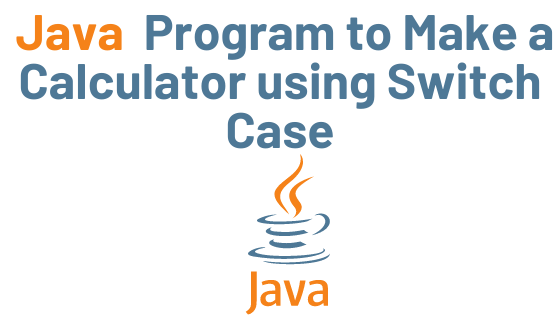## Java Program to Make a Calculator using Switch Case with Example

We are creating a simple calculator in this program that performs addition, subtraction, multiplication, and division based on user input. The program takes the value of both numbers (entered by the user) and then asks the user to enter the operation (+, -, *, and /), and based on the input, the program performs the selected operation on the entered numbers using a switch case.

## Example: To make a calculator using switch case in Java

``` import java.util.Scanner;

public class JavaExample {

public static void main(String[] args) {

double num1, num2;
Scanner scanner = new Scanner(System.in);
System.out.print("Enter first number:");

/* We are using data type double so that user
* can enter integer as well as floating point
* value
*/
num1 = scanner.nextDouble();
System.out.print("Enter second number:");
num2 = scanner.nextDouble();

System.out.print("Enter an operator (+, -, *, /): ");
char operator = scanner.next().charAt(0);

scanner.close();
double output;

switch(operator)
{
case '+':
output = num1 + num2;
break;

case '-':
output = num1 - num2;
break;

case '*':
output = num1 * num2;
break;

case '/':
output = num1 / num2;
break;

/* If user enters any other operator or char apart from
* +, -, * and /, then display an error message to user
*
*/
default:
System.out.printf("You have entered wrong operator");
return;
}

System.out.println(num1+" "+operator+" "+num2+": "+output);
}
}```

Output:

Enter first number:30
Enter second number:3
Enter an operator (+, -, *, /): /
30.0 / 3.0: 10.0